# 3. Find the positive root of In(x²) = 0.7 20-points a) Using three iterations of the...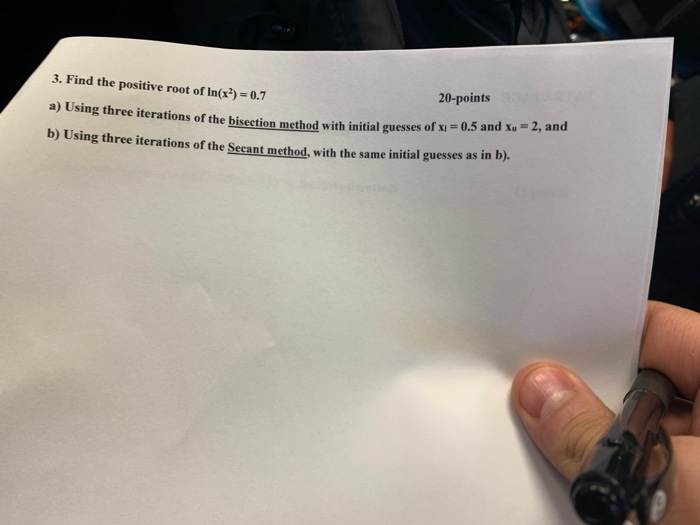3. Find the positive root of In(x²) = 0.7 20-points a) Using three iterations of the bisection method with initial guesses of Xi on method with initial guesses of x = 0.5 and Xu 2, and b) Using three iterations of the Secant method, with the same initial guesses as in .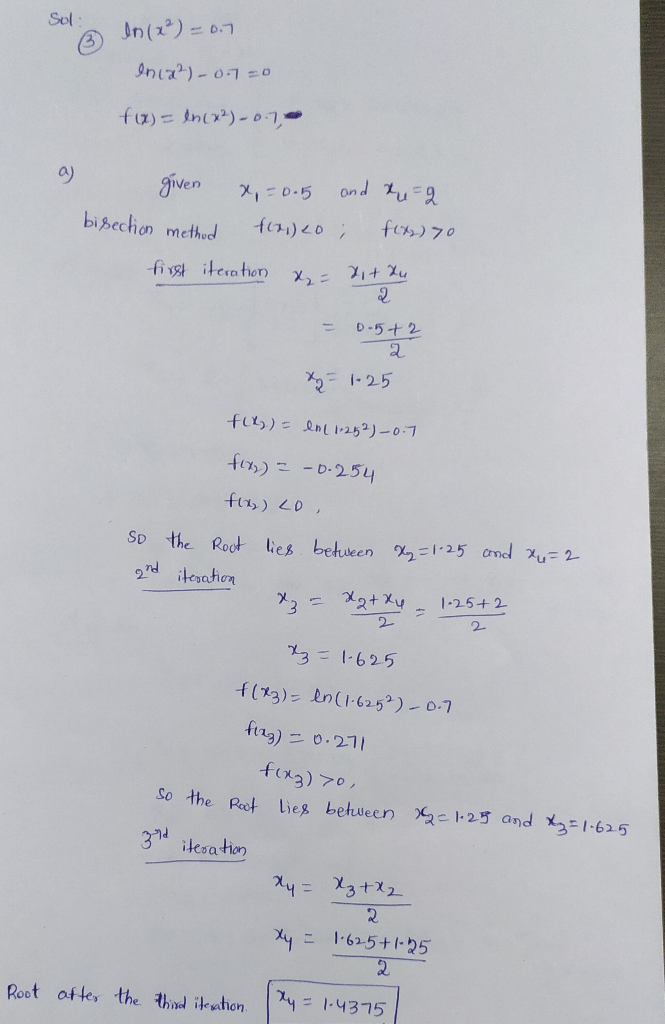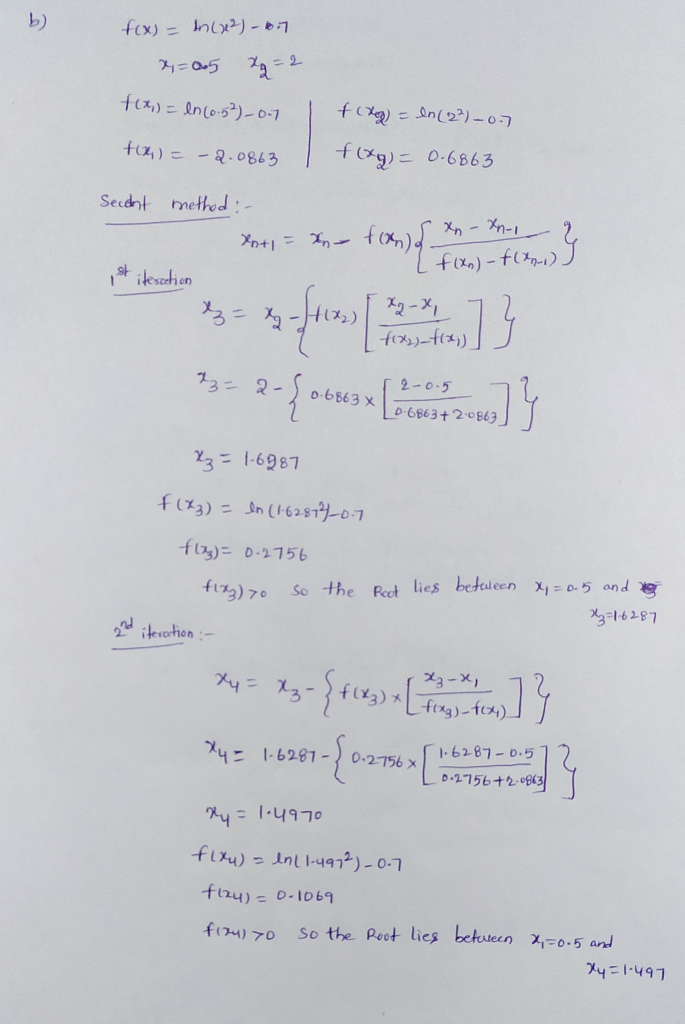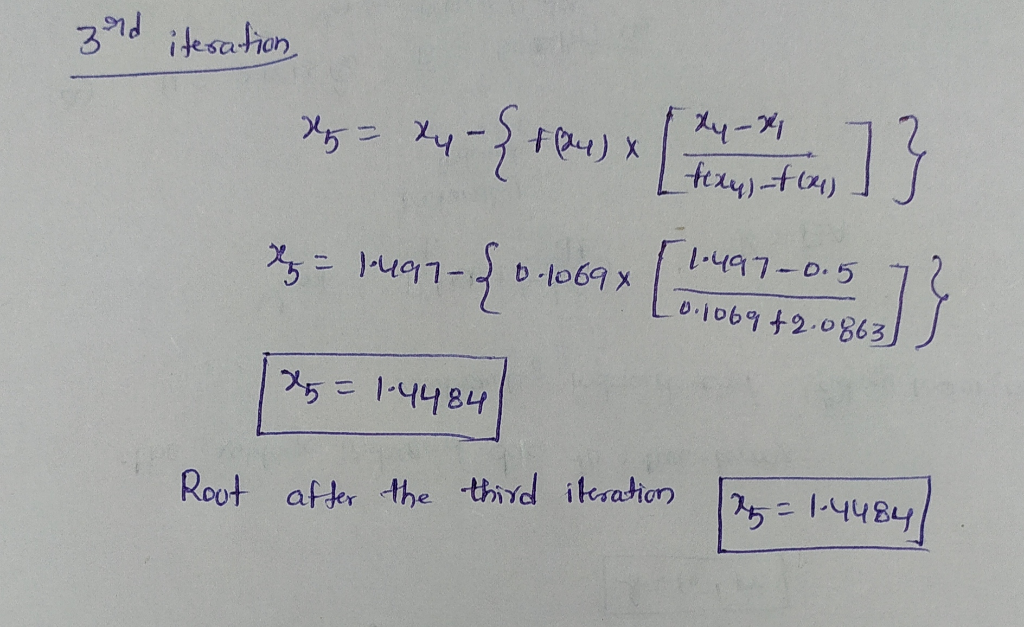#### Earn Coin

Coins can be redeemed for fabulous gifts.

Similar Homework Help Questions
• ### L. Determine the real root(s) off(x)--5xs + 14x3 + 20x2 + 10x a. Graphically on a graph paper. b....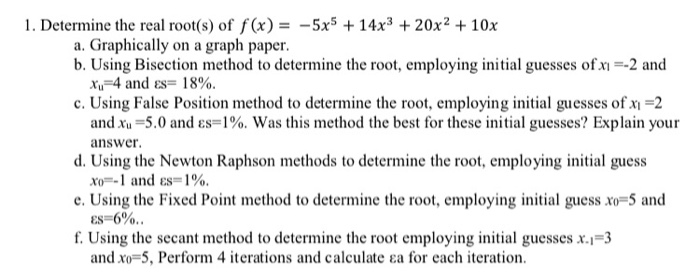l. Determine the real root(s) off(x)--5xs + 14x3 + 20x2 + 10x a. Graphically on a graph paper. b. Using Bisection method c. Using False Position method to determine the root, employing initial guesses of x-2 d. Using the Newton Raphson methods to determine the root, employing initial guess to determine the root, employing initial guesses ofxn-2 and Xu-4 and Es= 18%. and r 5.0 andas answer. 1%. was this method the best for these initial guesses? Explain your xo--l...

• ### lowest positive root of f(x)= 8sin(e-x)-1

Determine the lowest positive root of f (x) = 8sin(x)e–x – 1:(a) Graphically.(b) Using the Newton-Raphson method (three iterations, xi = 0.3).(c) Using the secant method (three iterations, xi–1 = 0.5 and xi = 0.4.(d) Using the modified secant method (five iterations, xi = 0.3, δ = 0.01).

• ### 6.5 Employ the Newton-Raphson method to determine a real root for 4x20.5 using initial guesses of...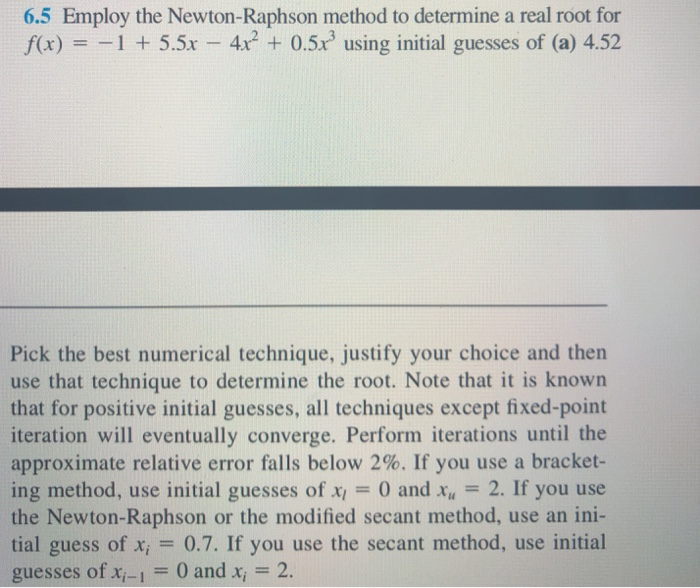6.5 Employ the Newton-Raphson method to determine a real root for 4x20.5 using initial guesses of (a) 4.52 f(x) 15.5x Pick the best numerical technique, justify your choice and then use that technique to determine the root. Note that it is known that for positive initial guesses, all techniques except fixed-point iteration will eventually converge. Perform iterations until the approximate relative error falls below 2 %. If you use a bracket- ing method, use initial guesses of x 0 and...

• ### 2) (15 points) a) Determine the roots of f(x)=-12 – 21x +18r? - 2,75x' graphically. In...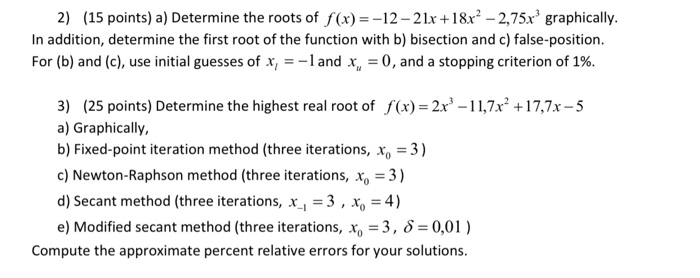2) (15 points) a) Determine the roots of f(x)=-12 – 21x +18r? - 2,75x' graphically. In addition, determine the first root of the function with b) bisection and c) false-position. For (b) and (c), use initial guesses of x, =-land x, = 0, and a stopping criterion of 1%. 3) (25 points) Determine the highest real root of f(x) = 2x – 11,7x² +17,7x-5 a) Graphically, b) Fixed-point iteration method (three iterations, x, = 3) c) Newton-Raphson method (three iterations,...

• ### 2. (40 points) Consider the following equation f(z) = 0.12,3-512-2+eT+4 (a) Plot the function in the...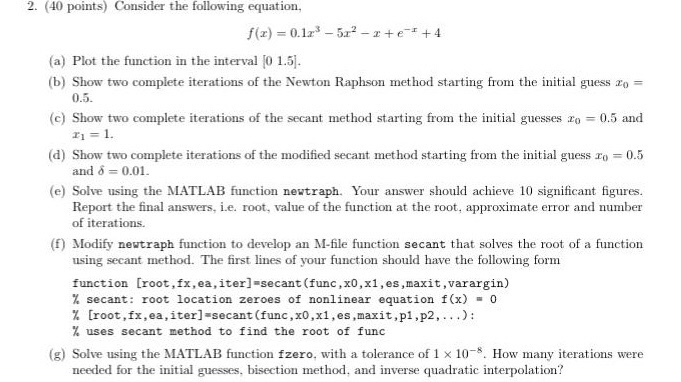2. (40 points) Consider the following equation f(z) = 0.12,3-512-2+eT+4 (a) Plot the function in the interval 0 1.5 (b) Show two complete iterations of the Newton Raphson method starting from the initial guess zo- 0.5 (c) Show two complete iterations of the secant method starting from the initial guesses zo 0.5 and (d) Show two complete iterations of the modified secant method starting from the initial guess o 0.5 (e) Solve using the MATLAB function newtraph. Your answer should...

• ### 1. 10 points Find the positive solution of the equation cosx=0.3x (ie. the positive root of...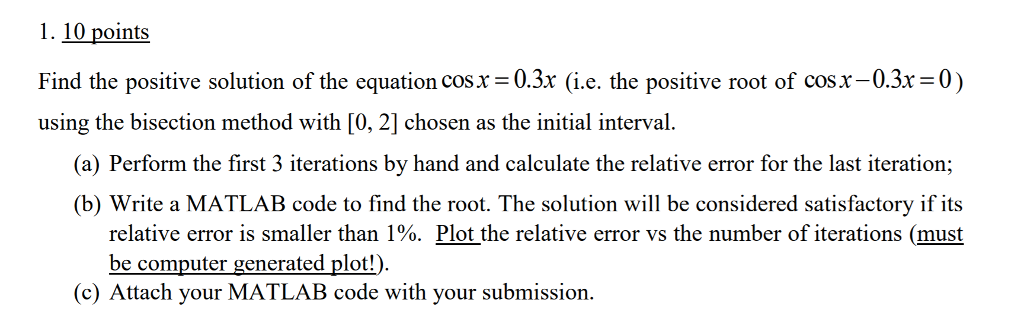1. 10 points Find the positive solution of the equation cosx=0.3x (ie. the positive root of cosx-03x=0) using the bisection method with [0, 2] chosen as the initial interval. (a) Perform the first 3 iterations by hand and calculate the relative error for the last iteration; (b) Write a MATLAB code to find the root. The solution will be considered satisfactory if its relative error is smaller than 1%. Plot the relative error vs the number of iterations (must be...

• ### PLEASE explain and show work? 5. (30 points) Use the secant method to find a root...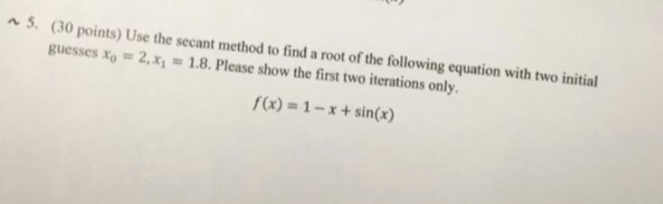PLEASE explain and show work? 5. (30 points) Use the secant method to find a root of the following equation with two initial guesses xo 2.x1 1.8. Please show the first two iterations only. f(x) = 1-x + sin(x)

• ### [20 Marks] Question 2 a) Given f(x)= x - 7x2 +14x-6 i) Show that there is...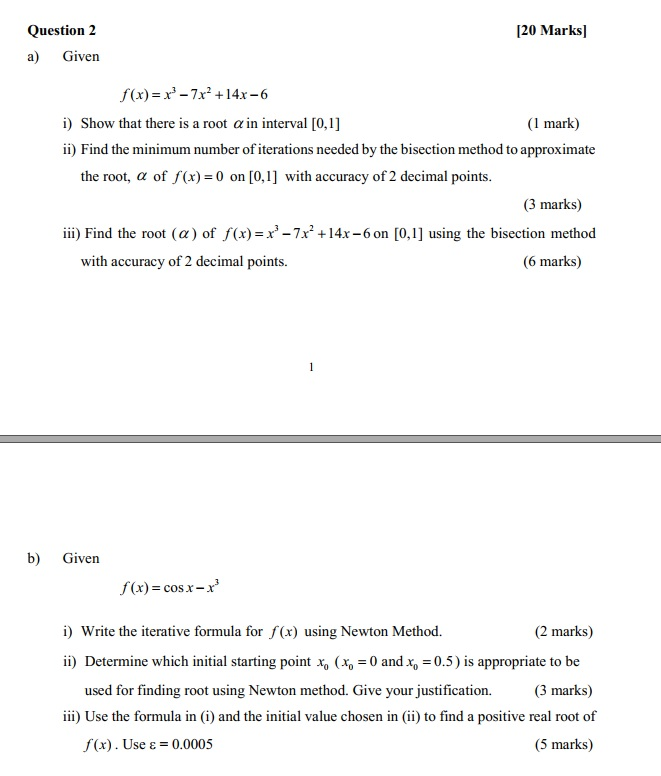[20 Marks] Question 2 a) Given f(x)= x - 7x2 +14x-6 i) Show that there is a root a in interval [0,1] (1 mark) ii) Find the minimum number of iterations needed by the bisection method to approximate the root, a of f(x) = 0 on [0,1] with accuracy of 2 decimal points. (3 marks) iii) Find the root (a) of f(x)= x - 7x² +14x6 on [0,1] using the bisection method with accuracy of 2 decimal points. (6 marks)...

• ### Problem 2 Determine the real roots of f(x)=-25 +82.x - 90x2 +44x-8x +0.7.x a) Graphically b)...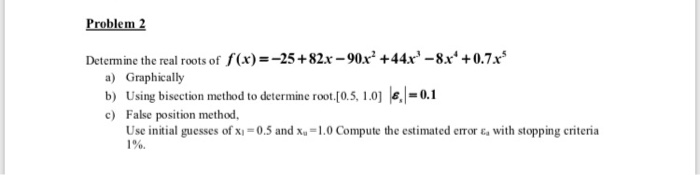Problem 2 Determine the real roots of f(x)=-25 +82.x - 90x2 +44x-8x +0.7.x a) Graphically b) Using bisection method to determine root (0.5, 1.0) 6 = 0.1 c) False position method, Use initial guesses of x=0.5 and x. -1.0 Compute the estimated errore, with stopping criteria 1%

• ### A5.2. Write a matlab program to find out the root of equation f (x)-x*-3x - 1, using false-positi...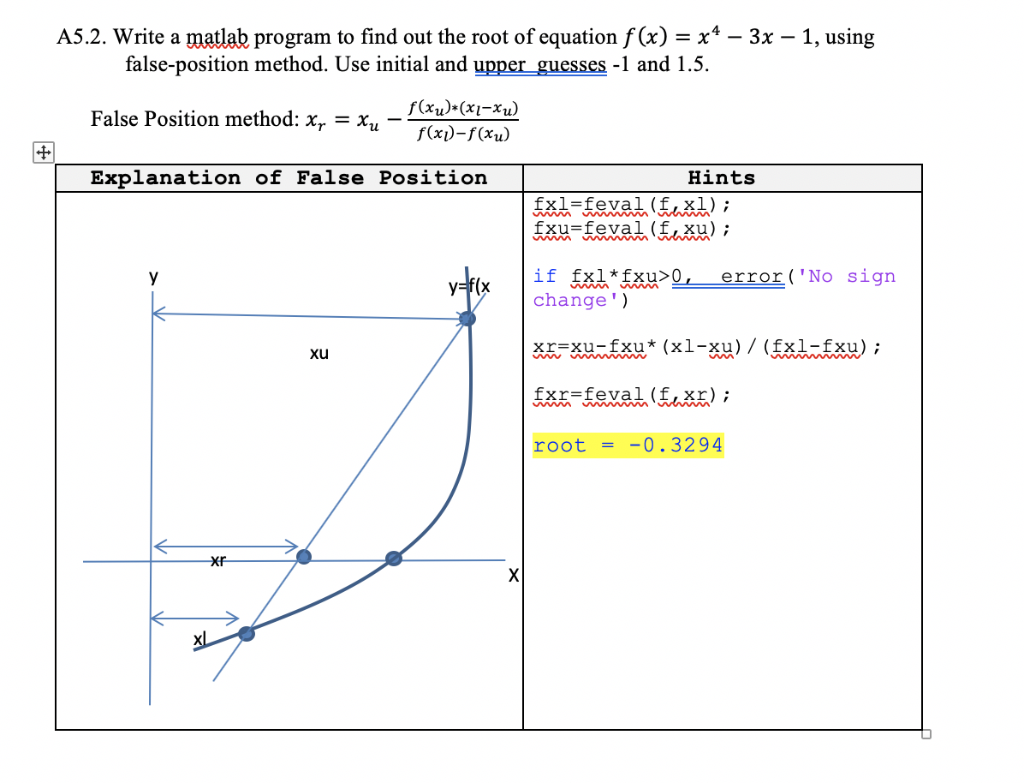numerical method lab A5.2. Write a matlab program to find out the root of equation f (x)-x*-3x - 1, using false-position method. Use initial and upper guesses -1 and 1.5 False Position method: xr-Xu Explanation of False Position f(x)(x-xu) Hints change) xu far-feval (foxr) ; root-0.3294 Xr A5.2. Write a matlab program to find out the root of equation f (x)-x*-3x - 1, using false-position method. Use initial and upper guesses -1 and 1.5 False Position method: xr-Xu Explanation of...

Free Homework Help App